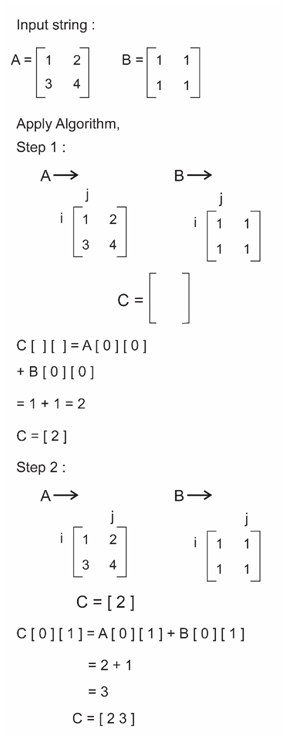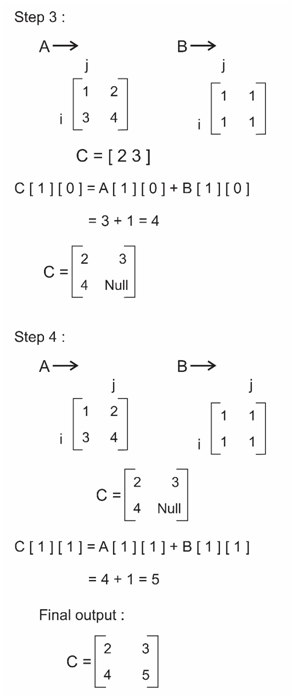## Given two matrices, we need to add the two matrices and give new matrix.

### Example

Input : A = { {1, 4, 7, 9},
{1, 6, 7, 6},
{6, 3, 7, 2},
{4, 4, 4, 4}}
B = { {1, 1, 1, 1},
{2, 2, 2, 2},
{3, 3, 3, 3},
{4, 4, 4, 4}}

Output : Let C = A + B
C = { {2, 5, 8, 10},
{3, 8, 9, 8},
{9, 6, 10, 5},
{8, 8, 8, 8}}

## Algorithm

Time Complexity : O(n^2)

Step 1 : For each row in the two matrices. (Let A and B be matrices we need to add)
a)    Add the respective elements in matrix A with the elements in matrix B.
b)    Store the result in same position in some auxiliary matrix b.
Step 2 : Move to next row and do the same.
Step 3 : Print the auxiliary matrix. (It is the sum of Aan B)

### Algorithm working## C++ Program

``````#include <bits/stdc++.h>
using namespace std;
#define N 4
int main()
{
int A[N][N] = { {1, 4, 7, 9},
{1, 6, 7, 6},
{6, 3, 7, 12},
{4, 4, 4, 4}};

int B[N][N] = { {1, 1, 1, 1},
{2, 2, 2, 2},
{3, 3, 3, 3},
{4, 9, 1, 7}};

int C[N][N]; //C = A + B
for(int i=0; i <N; i++)
{
for(int j=0; j<N; j++)
{
C[i][j] = A[i][j] + B[i][j];
cout<<A[i][j]<<" ";
}
cout<<endl;
}
for(int i=0; i <N; i++)
{
for(int j=0; j<N; j++)
{
cout<<C[i][j]<<" ";
}
cout<<endl;
}

return 0;
}``````

Scroll to Top